# GSEB Solutions Class 7 Maths Chapter 5 Lines and Angles Ex 5.2

Gujarat Board GSEB Textbook Solutions Class 7 Maths Chapter 5 Lines and Angles Ex 5.2 Textbook Questions and Answers.

## Gujarat Board Textbook Solutions Class 7 Maths Chapter 5 Lines and Angles Ex 5.2Question 1.
State the property that is used in each of the following statements?
(i) If a $$\parallel$$ b, then ∠1 = ∠5.
(ii) If ∠4 = ∠6, then a $$\parallel$$ b.
(iii) If ∠4 + ∠5 = 180°, then a $$\parallel$$ b.Solution:
(i) If two parallel lines are intersected by a transversal, then corresponding angles are equal.
(ii) If two given lines are intersected by a transversal such that interior alternate angles are equal, then the lines are parallel.
(iii) If two given lines are intersected by a transversal such that the sum of the interior angles on the same side of the transversal is 180°, then the lines are parallel.Question 2.
(i) the pairs of corresponding angles.
(ii) the pairs of alternate interior angles.
(iii) the pairs of interior angles on the same side of the transversal.
(iv) the vertically opposite angles.Solution:
(i) The pairs of corresponding angles are:
(∠1, ∠5); (∠2, ∠6); (∠3, ∠l) and (∠4, ∠8)

(ii) The pairs of alternate interior angles are:
(∠2, ∠8) and (∠3, ∠5)

(iii) The pairs of interior angles on the same side of the transversal are:
(∠2, ∠5) and (∠3, ∠8)

(iv) The vertically opposite angles are: .
(∠1, ∠3); (∠2, ∠4); (∠5, ∠7) and (∠6, ∠8)

Question 3.
In the adjoining figures p $$\parallel$$ g. Find the unknown angles.Solution:
Since, ∠e + 125° = 180°
[linear pair]
∴ ∠e = 180° – 125°
i.e. ∠e = 55°
∴ ∠e = ∠f
[Vertically opposite angles ]
∴ ∠f = 55° [∴ ∠e = 55°]
Also, ∠a =∠e [Corresponding angles]
∴ ∠a = 55°
Again ∠b = 125°
[Alternate exterior angles]
Since, ∠b and ∠c form a linear pair,
∴ ∠b + ∠c = 180° or 125° + ∠c = 180° or ∠c = 180° – 125° = 55°
Now, ∠b and ∠d are vertically opposite angles.
∴ ∠d = ∠b = 125° [∴ ∠b = 125°]
Thus, the required measures are:
∠a = 55°, ∠b = 125°, ∠c = 55°
∠d = 125°, ∠c = 55°, ∠f = 55°Question 4.
Find the value of x in each of the following figures if l || m.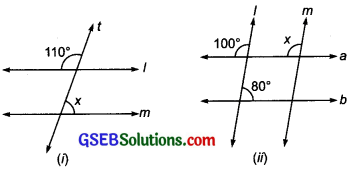Solution:
(i) l || m and t is transversal.∴ Alternate angles are equal, i.e. ∠x = ∠p
But ∠p + 110° = 180° [Linear pair]
or ∠p = 180° – 110° = 70°
∴ ∠x = 70° [∵ ∠p = ∠x]
Thus, the required value of x is 70°.

(ii) ∵ l and m are parallel and a is a transversal, I m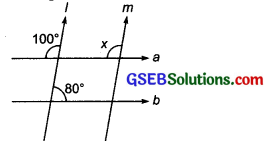∴ Corresponding angles are equal, i.e. ∠x = 100°
Thus, the required value of x is 100°.

Question 5.
In the given figure, the arms of two angles are parallel. If ∠ABC = 70°, then find:
(i) ∠DGC (ii) ∠DEF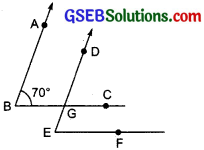Solution:
We have AB || ED and BC || EF
(i) BC is a transversal,
∴ ∠DGC = ∠ABC [Corresponding angles]
But ∠ABC = 70°
∴ ∠DGC = 70°

(ii) ED is a transversal to BC || EF
∴ ∠DEF = ∠DGC [Corresponding angles]
But ∠DGC = 70°
∴ ∠DEF = 70°Question 6.
In the given figures below, decide whether l is parallel to m.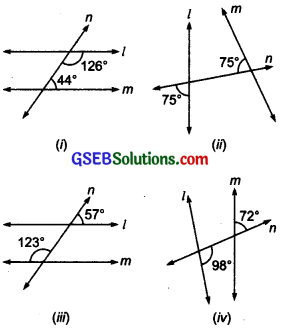Solution:
(i) ∵ 44° + 126° = 170°
But 170° ≠ 180°
i.e. the sum of the interior angles on the same side of the transversal is not 180°.
∴ l and m are not parallel.

(ii)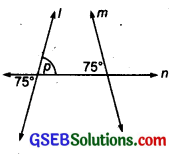∵ n is a transversal to l and m and ∠p = 75° [Vertically opposite angles]
Also ∠p + 75° = 75° + 75°
= 150°
And 150° ≠ 180°
∴ l and m are not parallel.

(iii)∴ ∠p + 123° = 180° [Linear pair]
∴ ∠p = 180° – 123°
= 57°
∴ ∠ p = 57°
i.e. corresponding angles are equal
∴ l and m are parallel.

(iv) ∵ ∠1 + ∠2 = 180° [Linear pair]
∴ ∠1 + 98° = 180°
or ∠1 = 180° – 98° = 82°
or ∠1 = 82°
Since ∠3 = 72°
∴ ∠1 ≠ ∠3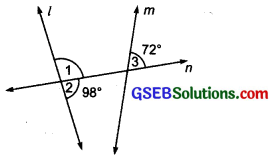i.e. the corresponding angles are not equal. Thus, l and m are not parallel.